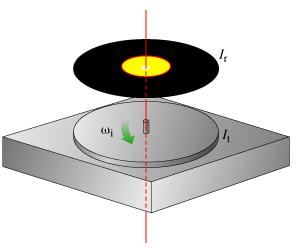# Problem: To understand how to use conservation of angular momentum to solve problems involving collisions of rotating bodies.Consider a turntable to be a circular disk of moment of inertia It rotating at a constant angular velocity ωi (note that angular velocities use the Greek letter omega and not double-u) around an axis through the center and perpendicular to the plane of the disk (the disk's "primary axis of symmetry") as shown in (Figure 1). 1. The axis of the disk is vertical and the disk is supported by frictionless bearings. The motor of the turntable is off, so there is no external torque being applied to the axis.Another disk (a record) is dropped onto the first such that it lands coaxially (the axes coincide). The moment of inertia of the record is Ir. The initial angular velocity of the second disk is zero.There is friction between the two disks.After this "rotational collision," the disks will eventually rotate with the same angular velocity.Part AWhat is the final angular velocity, ωf, of the two disks?Express ωf (omega subscript f) in terms of It, Ir, and ωi (omega subscript i).Part BBecause of friction, rotational kinetic energy is not conserved while the disks' surfaces slip over each other. What is the final rotational kinetic energy, Kf, of the two spinning disks?Express the final kinetic energy in terms of It, Ir, and the initial kinetic energy Ki of the two-disk system. No angular velocities should appear in your answer.

###### FREE Expert Solution

Part A

From the conservation of angular momentum,

$\begin{array}{rcl}\mathbf{\left(}{\mathbf{I}}_{\mathbf{t}}\mathbf{+}{\mathbf{I}}_{\mathbf{r}}\mathbf{\right)}{\mathbf{\omega }}_{\mathbf{f}}& \mathbf{=}& {\mathbf{I}}_{\mathbf{t}}{\mathbf{\omega }}_{\mathbf{i}}\\ {\mathbf{\omega }}_{\mathbf{f}}& \mathbf{=}& \frac{{\mathbf{I}}_{\mathbf{t}}{\mathbf{\omega }}_{\mathbf{i}}}{\mathbf{\left(}{\mathbf{I}}_{\mathbf{t}}\mathbf{+}{\mathbf{I}}_{\mathbf{r}}\mathbf{\right)}}\end{array}$

84% (156 ratings)###### Problem Details

To understand how to use conservation of angular momentum to solve problems involving collisions of rotating bodies.

Consider a turntable to be a circular disk of moment of inertia It rotating at a constant angular velocity ωi (note that angular velocities use the Greek letter omega and not double-u) around an axis through the center and perpendicular to the plane of the disk (the disk's "primary axis of symmetry") as shown in (Figure 1).

1. The axis of the disk is vertical and the disk is supported by frictionless bearings. The motor of the turntable is off, so there is no external torque being applied to the axis.

Another disk (a record) is dropped onto the first such that it lands coaxially (the axes coincide). The moment of inertia of the record is Ir. The initial angular velocity of the second disk is zero.

There is friction between the two disks.

After this "rotational collision," the disks will eventually rotate with the same angular velocity.Part A

What is the final angular velocity, ωf, of the two disks?

Express ωf (omega subscript f) in terms of ItIr, and ωi (omega subscript i).

Part B

Because of friction, rotational kinetic energy is not conserved while the disks' surfaces slip over each other. What is the final rotational kinetic energy, Kf, of the two spinning disks?

Express the final kinetic energy in terms of ItIr, and the initial kinetic energy Ki of the two-disk system. No angular velocities should appear in your answer.